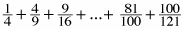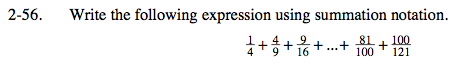### Home > PC > Chapter 2 > Lesson 2.2.2 > Problem2-56

2-56.

Write the following expression using summation notation. Homework Help ✎Count the number of terms.

10

Set up the sigma notation from 1 to 10.

$\displaystyle\sum\limits_{k=1}^{10}$

Complete the argument below.

$\displaystyle\sum\limits_{k=1}^{10}\frac{k^2}{(?)^2}$